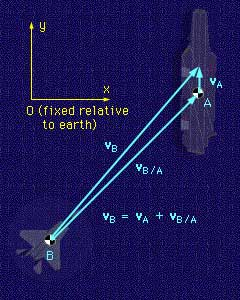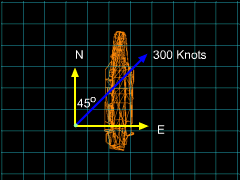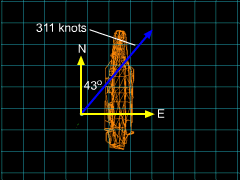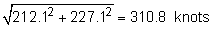Ch 1. Particle General Motion Multimedia Engineering Dynamics Position,Vel & Accel. Accel. varyw/ Time Accel. Constant Rect. Coordinates Norm/Tang. Coordinates Polar Coordinates RelativeMotion
 Chapter - Particle - 1. General Motion 2. Force & Accel. 3. Energy 4. Momentum - Rigid Body - 5. General Motion 6. Force & Accel. 7. Energy 8. Momentum 9. 3-D Motion 10. Vibrations Appendix Basic Math Units Basic Equations Sections Search eBooks Dynamics Fluids Math Mechanics Statics Thermodynamics Author(s): Kurt Gramoll ©Kurt GramollDYNAMICS - CASE STUDY SOLUTIONVelocity DirectionCarrier ViewEarth View Let the Nimitz be point A, and the F-15 be point B. The origin of the x-y coordinate system is fixed relative to the earth. The velocity of the Nimitz relative to the earth is      vA = 15j knots The velocity of the F-15 relative to the Nimitz is      vB/A = 300 cos45i + 300 sin45j knots Using the relative velocity equation, the velocity of the F-15 relative to the earth can be obtained as,      vB = vA + vB/A           = 300 cos45i + (15 + 300 sin45)j           = 212.1i + 227.1j The magnitude of the velocity of the F-15 relative to the earth isTrigonometry can be used to find the direction of the F-15 relative to the earth, giving      θ = tan-1 (212.1/227.1) = 43.0o East of North

Practice Homework and Test problems now available in the 'Eng Dynamics' mobile app
Includes over 400 problems with complete detailed solutions.
Available now at the Google Play Store and Apple App Store.